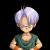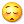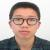正则表达式添加千位分隔符

my $number = <STDIN>; if($number =~ s/(\d{1,3})(?=(?:\d{3})+(?!\d)/$1,/g){ print$number;
}

s/(\d{1,3})(?=(?:\d{3})+(?!\d|$)/$1,/g

my $intNumber = int($number);
if ($number !=$intNumber){
$number =~ s/(\d{1,3})(?=(?:\d{3})+(?!(\d|$)))/$1,/g; print$number;
}else{
$number =~ s/(\d{1,3})(?=(?:\d{3})+(?!(\d)))/$1,/g;
print $number; } (?!(\d|$))可以解释为不是非数字或不是末尾,还是加上括号不容易歧义。

0看不懂
0